# EVM Measurement of 5G NR PUSCH Waveforms

This example shows how to measure the error vector magnitude (EVM) of PUSCH fixed reference channel (FRC) waveforms. The example also shows how to add impairments including phase noise and memoryless nonlinearity.

### Introduction

For base station receiver RF testing, the 3GPP 5G NR standard defines a set of FRC waveforms. The FRCs for frequency range 1 (FR1) and frequency range 2 (FR2) are defined in TS 38.104, Annex A.

This example shows how to generate an NR waveform (FRC) and add impairments. The example uses phase noise and memoryless nonlinearities. It shows how to calculate the EVM of the resulting signal, and then plot the root mean square (RMS) and peak EVMs per orthogonal frequency division multiplexing (OFDM) symbol, slot, and subcarrier. Then calculate the overall EVM (RMS EVM averaged over the complete waveform). Annex F of TS 38.101-1 and TS 38.101-2 define an alternative method for computing the EVM in FR1 and FR2, respectively. The figure below shows the processing chain implemented in this example.### Simulation parameters

Each FRC waveform is defined by a combination of these parameters:

• Frequency range number

• Order of the modulation and coding scheme (MCS) used

• Index of the FRC for the given MCS

Select one of the Release 15 FRCs for FR1 and FR2.

The selected reference channel (rc) must follow a G-FR X -A Y - Z format, where X is the frequency-range number (1 or 2), Y is the order of the MCS used in Annex A of TS 38.104 (i.e., a value from 1 to 5) and Z is the index of the FRC for the given MCS. The range of valid Z values depends on the frequency range and the MCS ( X and Y ) In case the input waveform is not a FRC waveform, atmost one layer is supported for EVM measurement.

```rc = "G-FR1-A1-7"; % FRC ```

To print EVM statistics, set `displayEVM` to `true`. To disable the prints, set `displayEVM` to `false`. To plot EVM statistics, set `plotEVM` to `true`. To disable the plots, set `plotEVM` to `false`

```displayEVM = true; plotEVM = true; ```
```if displayEVM fprintf('Reference Channel = %s\n',rc); end ```
```Reference Channel = G-FR1-A1-7 ```

To measure EVM as defined in TS 38.101-1 (FR1) or TS 38.101-2 (FR2), Annex F respectively, set `evm3GPP` to `true`. `evm3GPP` is disabled by default.

```evm3GPP = false; ```

Create a waveform generator object,then and generate the waveform.

```wavegen = hNRReferenceWaveformGenerator(rc); [txWaveform,tmwaveinfo,resourcesinfo] = generateWaveform(wavegen,wavegen.Config.NumSubframes); ```

### Impairment: Phase Noise and Memoryless Nonlinearity

This example considers two impairments: phase noise and memoryless nonlinearity. Enable or disable impairments by toggling the flags `phaseNoiseOn` and `nonLinearityModelOn`.

```phaseNoiseOn = true; nonLinearityModelOn = true; ```

Normalize the waveform to fit the dynamic range of the nonlinearity.

```txWaveform = txWaveform/max(abs(txWaveform),[],'all'); ```

The waveform consists of one frame for frequency division duplexing (FDD) and two for time division duplexing (TDD). Repeat the signal twice. For this example, remove the first half of the resulting waveform to avoid the transient introduced by the phase noise model.

```txWaveform = repmat(txWaveform,2,1); ```

Introduce phase noise distortion. The figure shows the phase noise characteristic. The carrier frequency considered depends on the frequency range. We use values of 4 GHz and 28 GHz for FR1 and FR2, respectively. The phase noise characteristic is generated with the pole or zero model described in R1-163984, "Discussion on phase noise modeling".

```if phaseNoiseOn sr = tmwaveinfo.Info.SamplingRate; % Carrier frequency if wavegen.Config.FrequencyRange == "FR1" % Carrier frequency for FR1 fc = 4e9; else % Carrier frequency for FR2 fc = 28e9; end % Calculate the phase noise level. foffsetLog = (3:0.1:log10(sr/2)); % Model offset from 1e3Hz to sr/2 foffset = 10.^foffsetLog; % Linear frequency offset PN_dBc_Hz = hPhaseNoisePoleZeroModel(foffset,fc,'A'); figure; semilogx(foffset,PN_dBc_Hz); xlabel('Frequency Offset (Hz)'); ylabel('dBc/Hz'); title('Phase noise model'); grid on % Apply phase noise to waveform pnoise = comm.PhaseNoise('FrequencyOffset',foffset,'Level',PN_dBc_Hz,'SampleRate',sr); rxWaveform = zeros(size(txWaveform),'like',txWaveform); for i = 1:size(txWaveform,2) rxWaveform(:,i) = pnoise(txWaveform(:,i)); release(pnoise) end else rxWaveform = txWaveform; %#ok<UNRCH> end ```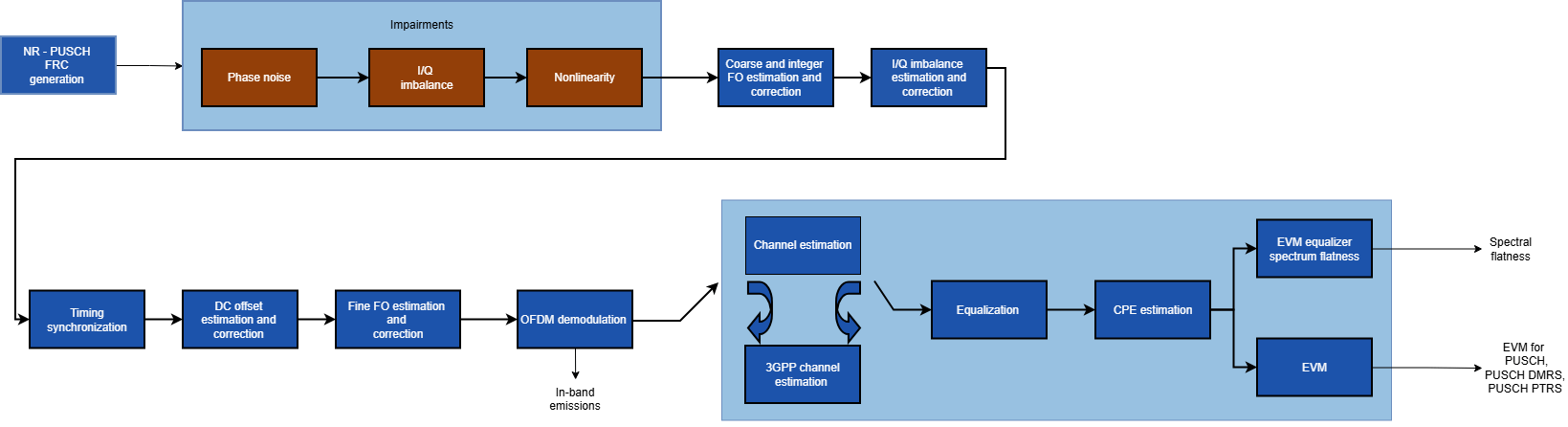Introduce nonlinear distortion. For this example, use the Rapp model. The figure shows the introduced nonlinearity. Set the parameters for the Rapp model to match the characteristics of the memoryless model from TR 38.803 "Memoryless polynomial model - Annex A.1".

```if nonLinearityModelOn rapp = comm.MemorylessNonlinearity('Method','Rapp model'); rapp.Smoothness = 1.55; rapp.OutputSaturationLevel = 1; % Plot nonlinear characteristic plotNonLinearCharacteristic(rapp); % Apply nonlinearity for i = 1:size(rxWaveform,2) rxWaveform(:,i) = rapp(rxWaveform(:,i)); release(rapp) end end ```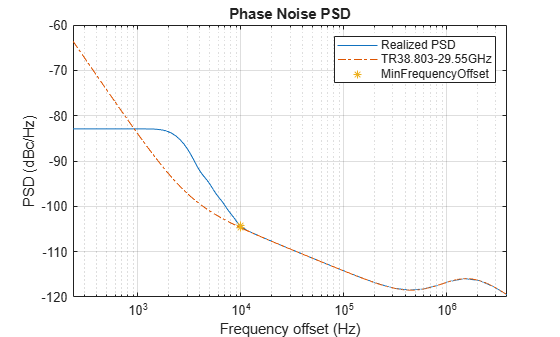The signal was previously repeated twice. Remove the first half of this signal to avoid any transient introduced by the impairment models.

```dm = wavegen.ConfiguredModel{4}; if dm == "FDD" nFrames = 1; else % TDD nFrames = 2; end rxWaveform(1:nFrames*tmwaveinfo.Info.SamplesPerSubframe*10,:) = []; ```

### Measurements

The function, hNRPUSCHEVM, performs these steps to decode and analyze the waveform:

• Coarse frequency offset estimation and correction

• Integer frequency offset estimation and correction

• Synchronization using the demodulation reference signal (DM-RS) over one frame for FDD (two frames for TDD)

• OFDM demodulation of the received waveform

• Fine frequency offset estimation and correction

• Channel estimation

• Equalization

• Common Phase error (CPE) estimation and compensation

• PUSCH EVM computation (enable the switch `evm3GPP` to process according to the EVM measurement requirements specified in TS 38.101-1(FR1) or TS 38.101-2 (FR2), Annex F.

The example measures and outputs various EVM related statistics per symbol, per slot, and per frame peak EVM and RMS EVM. The example displays the EVM for each slot and frame, and displays the overall EVM averaged over the entire input waveform. The example produces these plots: EVM versus per OFDM symbol, slot, subcarrier, overall EVM and in-band emissions. Each EVM related plot displays the peak versus RMS EVM.

```% Compute and display EVM measurements cfg = struct(); cfg.Evm3GPP = evm3GPP; cfg.PlotEVM = plotEVM; cfg.DisplayEVM = displayEVM; [evmInfo,eqSym,refSym] = hNRPUSCHEVM(wavegen.Config,rxWaveform,cfg); ```
```EVM stats for BWP idx : 1 RMS EVM, Peak EVM, slot 0: 2.170 7.345% RMS EVM, Peak EVM, slot 1: 2.001 5.759% RMS EVM, Peak EVM, slot 2: 2.038 6.248% RMS EVM, Peak EVM, slot 3: 2.040 6.352% RMS EVM, Peak EVM, slot 4: 2.108 5.690% RMS EVM, Peak EVM, slot 5: 2.039 5.747% RMS EVM, Peak EVM, slot 6: 2.234 6.997% RMS EVM, Peak EVM, slot 7: 2.176 7.760% RMS EVM, Peak EVM, slot 8: 2.128 7.049% RMS EVM, Peak EVM, slot 9: 2.460 7.097% Averaged RMS EVM frame 0: 2.143% Averaged overall RMS EVM: 2.143% Peak EVM : 7.7604% ```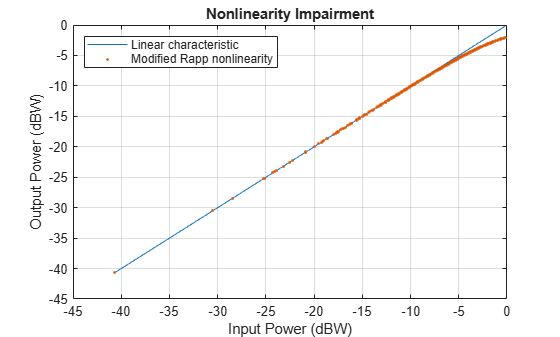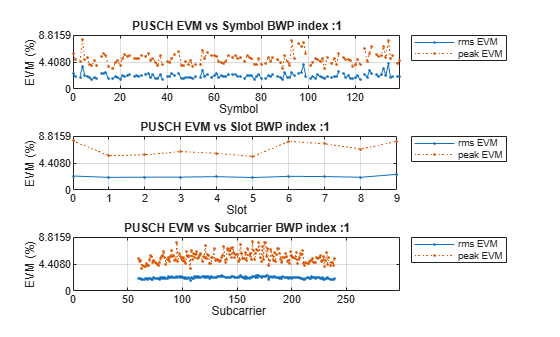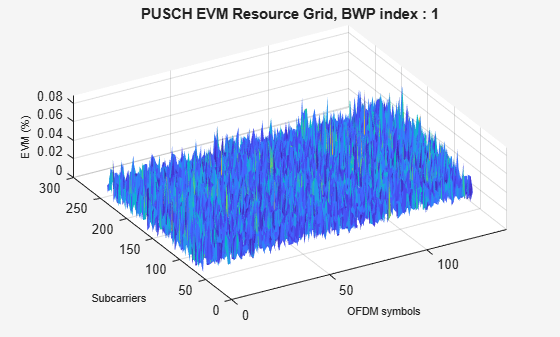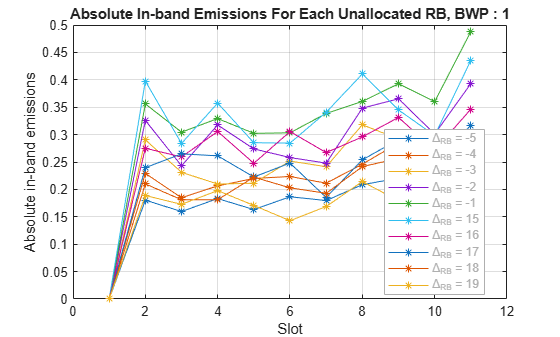### Local functions

```function plotNonLinearCharacteristic(memoryLessNonlinearity) % Plot the nonlinear characteristic of the power amplifier (PA) impairment % represented by the input parameter memoryLessNonlinearity, which is a % comm.MemorylessNonlinearity Communications Toolbox(TM) System object. % Input samples x = complex((1/sqrt(2))*(-1+2*rand(1000,1)),(1/sqrt(2))*(-1+2*rand(1000,1))); % Nonlinearity yRapp = memoryLessNonlinearity(x); % Release object to feed it a different number of samples release(memoryLessNonlinearity); % Plot characteristic figure; plot(10*log10(abs(x).^2),10*log10(abs(x).^2)); hold on; grid on plot(10*log10(abs(x).^2),10*log10(abs(yRapp).^2),'.'); xlabel('Input Power (dBW)'); ylabel('Output Power (dBW)'); title('Nonlinearity Impairment') legend('Linear characteristic', 'Rapp nonlinearity','Location','Northwest'); end ```